# Newton's diverging parabola

## cubic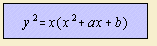Newton distinguished four classes of cubics. The diverging parabola is the third class. Newton states: 'in the third Case the Equation was yy = ax3 + bxx + cx + d; and defines a Parabola whose Legs diverge from one another, and run out infinitely contrary ways.'

These divergent parabolas can be divided in the following species, depending on the solution on the right hand side of the equation:

Newton distinguished five species, taking account of the two forms of Tschirnhausen's cubic.

## semicubic(al) parabola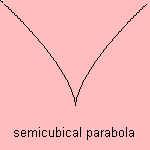As being a kind of a Newton's diverging parabola, it is the situation where the three real roots of the right hand equation are equal.

Its name is derived from the cubic parabola. The third (cubic) power is divided by two (semi).

An other name for the curve was derived from William Neil(e) (1637-1670) who discovered the curve in 1657: Neile's parabola. This very keen mathematician showed great promise, but the refusal by his father of the marriage with his beloved girl did break him. And he died young.
Newton said already (1710): 'this is the Neilian Parabola, commonly called Semi-Cubical'.

The curve was the first algebraic curve to have its arc length calculated. Congratulations! It was Wallis who published this calculation, in 1659; he gave the credits to his pupil, Neile.
The Dutch Van Heuraet used the curve for a more general construction.

In 1687 Leibniz challenged his fellow mathematicians with the question about the isochronous curve: under gravity, the vertical velocity of an object, rolling over this curve, is constant 1). In 1690 Jacob Bernoulli found that the given curve is the semi-cubic parabola (change x and y in above formula). Also Huygens found this solution.

You can also find this curve when considering an object as cause of the gravitation of, easy to imagine, the earth. The corresponding equation for the distance s(t) as function of the elapsed time t is s" = - g M / s ˛. It is easy to verify that the semicubic parabola t(s) is a solution, isn't it? 2).

The curve is the evolute of the parabola, and the catacaustic of Tschirnhausen's cubic.

## Newton's egg curve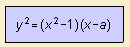Newton says (1710) about this case: 'then the figure is a diverging Parabola of the Form of a Bell, with an Oval at its vertex.'By some linear transformations the three variables corresponding to the roots can be reduced to one variable a.

Because the curve has the form of an egg, I use the name of Newton's egg curve.

## Tschirnhaus(en)'s cubic

or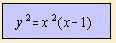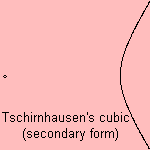Two of the three real roots are equal. The curve has two forms, the first is more common in curves' literature. The origin in above formulae is called the 'pole' of the curve.

Newton says (1710) about the curve: 'a Parabola will be formed, either Nodated by touching an Oval, or Punctate, by having the Oval infinitely small'.
Later on, the curve was studied by Tschirnhaus(en) (in 1690), de L'Hôpital (in 1696) and Catalan. So that for the curve the following names can be found: Tschirnhaus(en)'s cubic, (de) L'Hôpital's cubic and trisectrix of Catalan.
It was R.C. Archibald who gave the curve its Tschirnhausen’s cubic name, in a paper written in 1900.

The curve can also be seen as a sinusoidal spiral (a=1/3) 3).

Some interesting properties of the curve are:

The curve has as polar equation the form r = cos-3φ and she is also called the cubic duplicatrix.

## one real root

There is just one real root: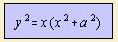Newton said in 1710: 'if two of the roots are impossible, there will be a Pure Parabola of a Bell-like Form'.

notes

1) the problem is solved by substituting ds/dt in:and dividing numerator and denominator by dx.

2) Walter 1976 p. 4.

3) You can transfer the spiral equation to the Cartesian equation using f = 3 / tan t.